## Example Questions

### Example Question #164 : Plane Geometry

Two interior angles in an obtuse triangle measure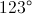and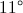. What is the measurement of the third angle.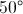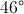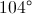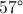Explanation:

Interior angles of a triangle always add up to 180 degrees.

### Example Question #165 : Plane Geometry

In a given triangle, the angles are in a ratio of 1:3:5.  What size is the middle angle?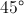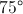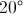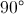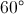Explanation:

Since the sum of the angles of a triangle is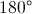, and given that the angles are in a ratio of 1:3:5, let the measure of the smallest angle be, then the following expression could be written: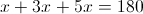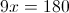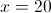If the smallest angle is 20 degrees, then given that the middle angle is in ratio of 1:3, the middle angle would be 3 times as large, or 60 degrees.

### Example Question #166 : Plane Geometry

In the triangle below, AB=BC (figure is not to scale) .  If angle A is 41°, what is the measure of angle B?

A (Angle A = 41°)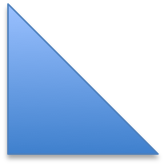B                           C

82

98

41

90

98

Explanation:

If angle A is 41°, then angle C must also be 41°, since AB=BC.  So, the sum of these 2 angles is:

41° + 41° = 82°

Since the sum of the angles in a triangle is 180°, you can find out the measure of the remaining angle by subtracting 82 from 180:

180° - 82° = 98°

### Example Question #171 : Plane Geometry

Points A, B, C, D are collinear. The measure of ∠ DCE is 130° and of ∠ AEC is 80°. Find the measure of ∠ EAD.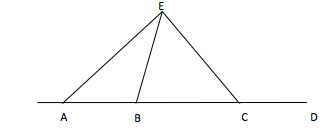70°

80°

60°

50°

50°

Explanation:

To solve this question, you need to remember that the sum of the angles in a triangle is 180°. You also need to remember supplementary angles. If you know what ∠ DCE is, you also know what ∠ ECA is. Hence you know two angles of the triangle, 180°-80°-50°= 50°.

### Example Question #2 : How To Find An Angle In An Acute / Obtuse Triangle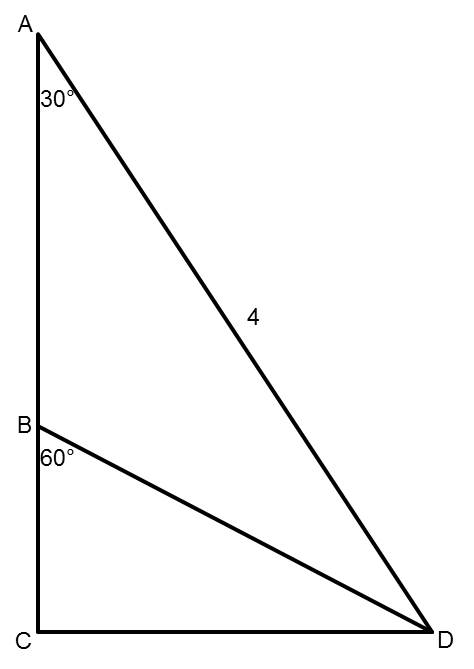Points A, B, and C are collinear (they lie along the same line). The measure of angle CAD is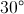. The measure of angle CBD is. The length of segment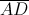is 4.

Find the measure of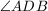.The measure ofis. Since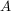,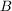, and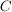are collinear, and the measure of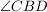is, we know that the measure of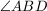is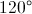.
Because the measures of the three angles in a triangle must add up to, and two of the angles in triangle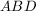areand, the third angle,, is.